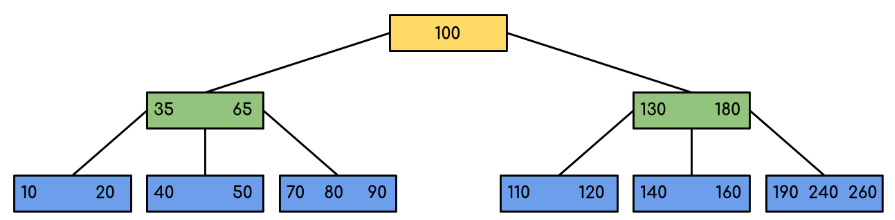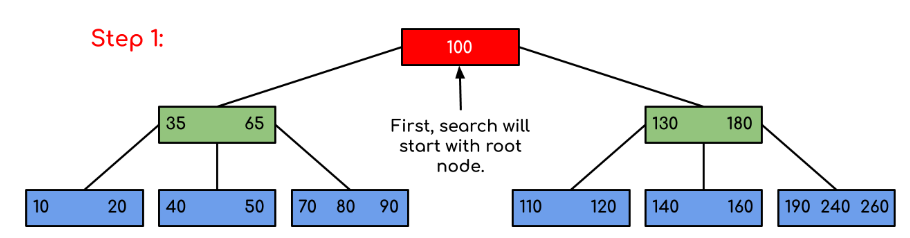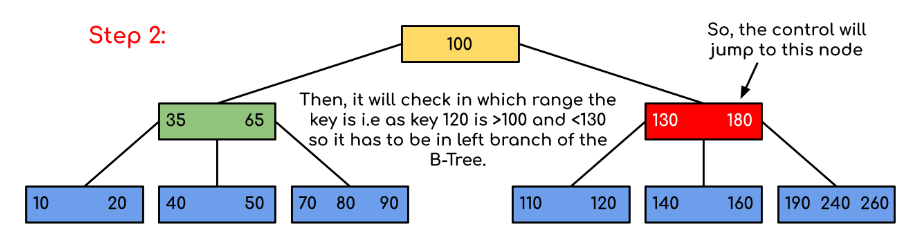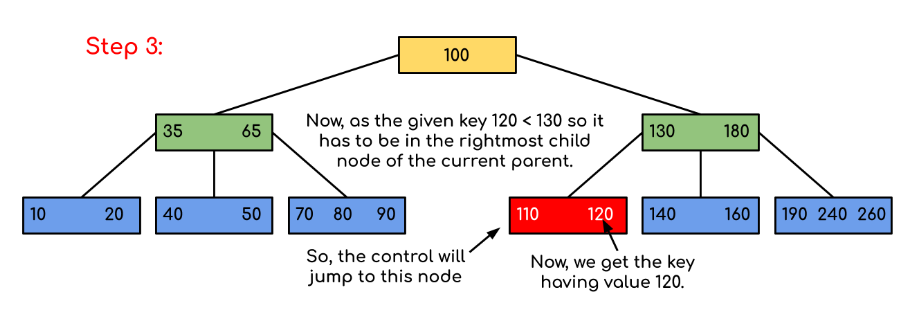# Introduction of B-Tree

• Difficulty Level : Hard
• Last Updated : 01 Sep, 2022

B-Tree is a self-balancing search tree. In most of the other self-balancing search trees (like AVL and Red-Black Trees), it is assumed that everything is in the main memory.

To understand the use of B-Trees, we must think of the huge amount of data that cannot fit in the main memory. When the number of keys is high, the data is read from the disk in the form of blocks. Disk access time is very high compared to the main memory access time. The main idea of using B-Trees is to reduce the number of disk accesses. Most of the tree operations (search, insert, delete, max, min, ..etc ) require O(h) disk accesses where h is the height of the tree. B-tree is a fat tree. The height of B-Trees is kept low by putting the maximum possible keys in a B-Tree node. Generally, the B-Tree node size is kept equal to the disk block size. Since the height of the B-tree is low so total disk accesses for most of the operations are reduced significantly compared to balanced Binary Search Trees like AVL Tree, Red-Black Tree, etc.

## Time Complexity of B-Tree:

Note: “n” is the total number of elements in the B-tree

## Properties of B-Tree:

• All leaves are at the same level.
• B-Tree is defined by the term minimum degree ‘t‘. The value of ‘t‘ depends upon disk block size.
• Every node except the root must contain at least t-1 keys. The root may contain a minimum of 1 key.
• All nodes (including root) may contain at most (2*t – 1) keys.
• Number of children of a node is equal to the number of keys in it plus 1.
• All keys of a node are sorted in increasing order. The child between two keys k1 and k2 contains all keys in the range from k1 and k2.
• B-Tree grows and shrinks from the root which is unlike Binary Search Tree. Binary Search Trees grow downward and also shrink from downward.
• Like other balanced Binary Search Trees, the time complexity to search, insert and delete is O(log n).
• Insertion of a Node in B-Tree happens only at Leaf Node.

Following is an example of a B-Tree of minimum order 5
Note: that in practical B-Trees, the value of the minimum order is much more than 5.We can see in the above diagram that all the leaf nodes are at the same level and all non-leafs have no empty sub-tree and have keys one less than the number of their children.

## Interesting Facts about B-Trees:

• The minimum height of the B-Tree that can exist with n number of nodes and m is the maximum number of children of a node can have is:• The maximum height of the B-Tree that can exist with n number of nodes and t is the minimum number of children that a non-root node can have is:and## Traversal in B-Tree:

Traversal is also similar to Inorder traversal of Binary Tree. We start from the leftmost child, recursively print the leftmost child, then repeat the same process for the remaining children and keys. In the end, recursively print the rightmost child.

## Search Operation in B-Tree:

Search is similar to the search in Binary Search Tree. Let the key to be searched is k.

• Start from the root and recursively traverse down.
• For every visited non-leaf node,
• If the node has the key, we simply return the node.
• Otherwise, we recur down to the appropriate child (The child which is just before the first greater key) of the node.
• If we reach a leaf node and don’t find k in the leaf node, then return NULL.

Searching a B-Tree is similar to searching a binary tree. The algorithm is similar and goes with recursion. At each level, the search is optimized as if the key value is not present in the range of the parent then the key is present in another branch. As these values limit the search they are also known as limiting values or separation values. If we reach a leaf node and don’t find the desired key then it will display NULL.

## C

 BtreeSearch(x, k)      i = 1             // n[x] means number of keys in x node      while i ≤ n[x] and k ≥ keyi[x]          do i = i + 1      if i  n[x] and k = keyi[x]          then return (x, i)         if leaf [x]          then return NIL      else         return BtreeSearch(ci[x], k)

Examples:

Input: Search 120 in the given B-Tree.Solution:In this example, we can see that our search was reduced by just limiting the chances where the key containing the value could be present. Similarly if within the above example we’ve to look for 180, then the control will stop at step 2 because the program will find that the key 180 is present within the current node. And similarly, if it’s to seek out 90 then as 90 < 100 so it’ll go to the left subtree automatically, and therefore the control flow will go similarly as shown within the above example.

Below is the implementation of the above approach:

## C++

 // C++ implementation of search() and traverse() methods  #include  using namespace std;     // A BTree node  class BTreeNode {      int* keys; // An array of keys      int t; // Minimum degree (defines the range for number             // of keys)      BTreeNode** C; // An array of child pointers      int n; // Current number of keys      bool leaf; // Is true when node is leaf. Otherwise false  public:      BTreeNode(int _t, bool _leaf); // Constructor         // A function to traverse all nodes in a subtree rooted      // with this node      void traverse();         // A function to search a key in the subtree rooted with      // this node.      BTreeNode*      search(int k); // returns NULL if k is not present.         // Make the BTree friend of this so that we can access      // private members of this class in BTree functions      friend class BTree;  };     // A BTree  class BTree {      BTreeNode* root; // Pointer to root node      int t; // Minimum degree  public:      // Constructor (Initializes tree as empty)      BTree(int _t)      {          root = NULL;          t = _t;      }         // function to traverse the tree      void traverse()      {          if (root != NULL)              root->traverse();      }         // function to search a key in this tree      BTreeNode* search(int k)      {          return (root == NULL) ? NULL : root->search(k);      }  };     // Constructor for BTreeNode class  BTreeNode::BTreeNode(int _t, bool _leaf)  {      // Copy the given minimum degree and leaf property      t = _t;      leaf = _leaf;         // Allocate memory for maximum number of possible keys      // and child pointers      keys = new int[2 * t - 1];      C = new BTreeNode*[2 * t];         // Initialize the number of keys as 0      n = 0;  }     // Function to traverse all nodes in a subtree rooted with  // this node  void BTreeNode::traverse()  {      // There are n keys and n+1 children, traverse through n      // keys and first n children      int i;      for (i = 0; i < n; i++) {          // If this is not leaf, then before printing key[i],          // traverse the subtree rooted with child C[i].          if (leaf == false)              C[i]->traverse();          cout << " " << keys[i];      }         // Print the subtree rooted with last child      if (leaf == false)          C[i]->traverse();  }     // Function to search key k in subtree rooted with this node  BTreeNode* BTreeNode::search(int k)  {      // Find the first key greater than or equal to k      int i = 0;      while (i < n && k > keys[i])          i++;         // If the found key is equal to k, return this node      if (keys[i] == k)          return this;         // If the key is not found here and this is a leaf node      if (leaf == true)          return NULL;         // Go to the appropriate child      return C[i]->search(k);  }

## Java

 // Java program to illustrate the sum of two numbers     // A BTree  class Btree {      public BTreeNode root; // Pointer to root node      public int t; // Minimum degree         // Constructor (Initializes tree as empty)      Btree(int t)      {          this.root = null;          this.t = t;      }         // function to traverse the tree      public void traverse()      {          if (this.root != null)              this.root.traverse();          System.out.println();      }         // function to search a key in this tree      public BTreeNode search(int k)      {          if (this.root == null)              return null;          else             return this.root.search(k);      }  }     // A BTree node  class BTreeNode {      int[] keys; // An array of keys      int t; // Minimum degree (defines the range for number             // of keys)      BTreeNode[] C; // An array of child pointers      int n; // Current number of keys      boolean         leaf; // Is true when node is leaf. Otherwise false         // Constructor      BTreeNode(int t, boolean leaf)      {          this.t = t;          this.leaf = leaf;          this.keys = new int[2 * t - 1];          this.C = new BTreeNode[2 * t];          this.n = 0;      }         // A function to traverse all nodes in a subtree rooted      // with this node      public void traverse()      {             // There are n keys and n+1 children, traverse          // through n keys and first n children          int i = 0;          for (i = 0; i < this.n; i++) {                 // If this is not leaf, then before printing              // key[i], traverse the subtree rooted with              // child C[i].              if (this.leaf == false) {                  C[i].traverse();              }              System.out.print(keys[i] + " ");          }             // Print the subtree rooted with last child          if (leaf == false)              C[i].traverse();      }         // A function to search a key in the subtree rooted with      // this node.      BTreeNode search(int k)      { // returns NULL if k is not present.             // Find the first key greater than or equal to k          int i = 0;          while (i < n && k > keys[i])              i++;             // If the found key is equal to k, return this node          if (keys[i] == k)              return this;             // If the key is not found here and this is a leaf          // node          if (leaf == true)              return null;             // Go to the appropriate child          return C[i].search(k);      }  }

## C#

 // C# program to illustrate the sum of two numbers  using System;     // A BTree  class Btree {      public BTreeNode root; // Pointer to root node      public int t; // Minimum degree         // Constructor (Initializes tree as empty)      Btree(int t)      {          this.root = null;          this.t = t;      }         // function to traverse the tree      public void traverse()      {          if (this.root != null)              this.root.traverse();          Console.WriteLine();      }         // function to search a key in this tree      public BTreeNode search(int k)      {          if (this.root == null)              return null;          else             return this.root.search(k);      }  }     // A BTree node  class BTreeNode {      int[] keys; // An array of keys      int t; // Minimum degree (defines the range for number             // of keys)      BTreeNode[] C; // An array of child pointers      int n; // Current number of keys      bool leaf; // Is true when node is leaf. Otherwise false         // Constructor      BTreeNode(int t, bool leaf)      {          this.t = t;          this.leaf = leaf;          this.keys = new int[2 * t - 1];          this.C = new BTreeNode[2 * t];          this.n = 0;      }         // A function to traverse all nodes in a subtree rooted      // with this node      public void traverse()      {             // There are n keys and n+1 children, traverse          // through n keys and first n children          int i = 0;          for (i = 0; i < this.n; i++) {                 // If this is not leaf, then before printing              // key[i], traverse the subtree rooted with              // child C[i].              if (this.leaf == false) {                  C[i].traverse();              }              Console.Write(keys[i] + " ");          }             // Print the subtree rooted with last child          if (leaf == false)              C[i].traverse();      }         // A function to search a key in the subtree rooted with      // this node.      public BTreeNode search(int k)      { // returns NULL if k is not present.             // Find the first key greater than or equal to k          int i = 0;          while (i < n && k > keys[i])              i++;             // If the found key is equal to k, return this node          if (keys[i] == k)              return this;             // If the key is not found here and this is a leaf          // node          if (leaf == true)              return null;             // Go to the appropriate child          return C[i].search(k);      }  }     // This code is contributed by Rajput-Ji

## Javascript

 // Javascript program to illustrate the sum of two numbers     // A BTree  class Btree  {         // Constructor (Initializes tree as empty)      constructor(t)      {          this.root = null;          this.t = t;      }             // function to traverse the tree      traverse()      {          if (this.root != null)              this.root.traverse();          document.write("
");      }             // function to search a key in this tree      search(k)      {          if (this.root == null)              return null;          else             return this.root.search(k);      }         }     // A BTree node  class BTreeNode  {       // Constructor      constructor(t,leaf)      {          this.t = t;          this.leaf = leaf;          this.keys = new Array(2 * t - 1);          this.C = new Array(2 * t);          this.n = 0;      }      // A function to traverse all nodes in a subtree rooted with this node      traverse()      {          // There are n keys and n+1 children, traverse through n keys          // and first n children          let i = 0;          for (i = 0; i < this.n; i++) {                  // If this is not leaf, then before printing key[i],              // traverse the subtree rooted with child C[i].              if (this.leaf == false) {                  C[i].traverse();              }              document.write(keys[i] + " ");          }              // Print the subtree rooted with last child          if (leaf == false)              C[i].traverse();      }             // A function to search a key in the subtree rooted with this node.      search(k)    // returns NULL if k is not present.      {                 // Find the first key greater than or equal to k          let i = 0;          while (i < n && k > keys[i])              i++;              // If the found key is equal to k, return this node          if (keys[i] == k)              return this;              // If the key is not found here and this is a leaf node          if (leaf == true)              return null;              // Go to the appropriate child          return C[i].search(k);      }  }     // This code is contributed by patel2127

Note: The above code doesn’t contain the driver program. We will be covering the complete program in our next post on B-Tree Insertion.

There are two conventions to define a B-Tree, one is to define by minimum degree, second is to define by order. We have followed the minimum degree convention and will be following the same in coming posts on B-Tree. The variable names used in the above program are also kept the same

## Applications of B-Trees:

• It is used in large databases to access data stored on the disk
• Searching for data in a data set can be achieved in significantly less time using the B-Tree
• With the indexing feature, multilevel indexing can be achieved.
• Most of the servers also use the B-tree approach.

Insertion and Deletion:
B-Tree Insertion
B-Tree Deletion

Please write comments if you find anything incorrect, or you want to share more information about the topic discussed above.

My Personal Notes arrow_drop_up
Recommended Articles
Page :